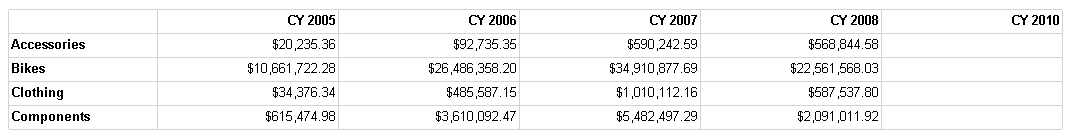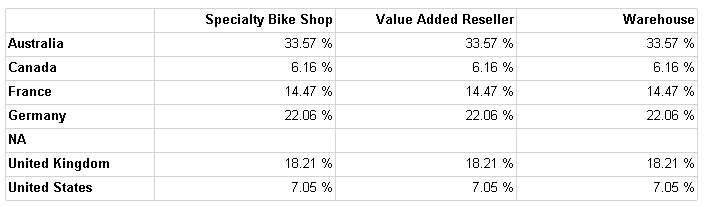In this post, I continue my exploration of approaches to working with dynamic MDX in a Reporting Services dataset when you are using Analysis Services as a data source. I began this series with a look at string conversion functions in Part 1. In Part 1, the dynamic MDX relied on parameters that work as a filter on the query results.

But what if you want to change the structure of the query itself? A parameter won’t help with that. Instead, you need to create the query string at run-time. However, the Analysis Services data source in Reporting Services doesn’t allow you to use an expression to define the query string. That’s okay- I’ll just do an end run around that problem. I’ll use an OLE DB provider to connect to my cube and then I can build up the query string by using an expression. In this post, I walk you through the process.

The context for this demonstration is a report that allows the user to specify the sets that appear on rows and columns of a matrix and to select one measure. It’s a very simple example that focuses on the dataset construction, and doesn’t spend as much time on the beautification of the report. Hopefully, it will give you some ideas to leverage for your own reports.

Create a data source

First, I need to create an OLE DB source. In the Type drop-down list, I select “OLE DB” and then I provide a connection string like this:

`Provider=MSOLAP.4;Data Source=.;Initial Catalog="Adventure Works DW 2008R2"`

The Edit button allows you to use a UI to generate the string if you don’t want to remember how to construct it manually.

Parameters

This set of steps is necessary to create the lists from which the user makes the selection. In my very simple example, I have created two parameters – Rows and Columns – and hard-coded possible lists. You can do more interesting things here, of course. Just make sure that the user can’t make the same selection for both parameters – whether you enforce that by manually providing the values or by doing something clever with a dynamically generated list based on a query.

I created the Measure parameter with the following values:

 Label Value Sales Amount =”[Measures].[Sales Amount]” Order Quantity =”[Measures].[Order Quantity]” Gross Profit Margin =”[Measures].[Gross Profit Margin]”

I created the Columns parameters like this:

And the Rows parameters like this:

 Label Value Product Category =”[Product].[Category].[Category].Members” Sales Territory Country =”[Sales Territory].[Sales Territory Country].[Sales Territory Country].Members”

Dataset

Although the ultimate goal is to produce a dynamic MDX query, it’s actually easier to start the design of the dataset using a static query and then to switch it out later. By using a static query, the fields for the dataset are autogenerated. The less work I have to do, the better.

Now one thing about dynamic MDX in Reporting Services is the need to make sure the number of fields in the dataset are the same each and every time. Therefore I need to structure the query differently than I would if I were to write it for a “normal” Analysis Services client. That is, I need to rewrite a query like this:

`select`
`non empty [Date].[Calendar Year].[Calendar Year].Members on columns,`
`non empty [Product].[Category].[Category].Members on rows`
`from [Adventure Works]`
`where [Measures].[Sales Amount]`
which produces a result like this: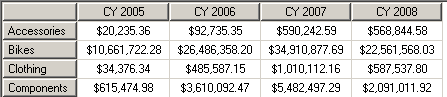to a query that looks like this:
`select`
```[Measures].[Sales Amount] on columns,
non empty
([Product].[Category].[Category].Members,
[Date].[Calendar Year].[Calendar Year].Members)
on rows

and produces a result like this: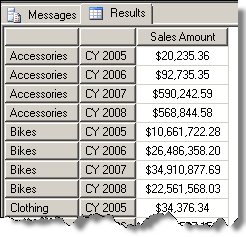However, while structurally the result set is what I need, the fields generated for the dimensions above will change each time that I run the dynamic query with different specifications for rows and columns. So I need to modify the query one more time like this:
```with
member [Measures].[Measure] as [Measures].[Sales Amount]
member [Measures].[RowValue] as [Product].[Category].CurrentMember.Name
member [Measures].[ColumnValue] as [Date].[Calendar Year].CurrentMember.Name
select {[Measures].[Measure], [Measures].[RowValue], [Measures].[ColumnValue]} on columns,
non empty ([Product].[Category].[Category].Members, [Date].[Calendar Year].[Calendar Year].Members) on rows

To get a result like this:Now I can reference the generic Measure, RowValue, and ColumnValue in the report layout.

Matrix

I like to test things out before I start introducing more complexity. So my next step is to add a matrix layout to the report design, and put fields into the layout and apply a little formatting as shown below.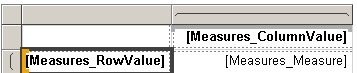One extra step related to formatting is required in my example. I have three possible measures, each of which uses a different format string. If I were displaying detail records, I could use the formatted_value cell property as an extended property in the textbox expression, replacing Fields!Measure.Value with Fields!Measure.FormattedValue. However, the use of a matrix here doesn’t work with that approach, so… I need to create a conditional expression to set the Format property correctly:

`=Switch(Parameters!Measure.Label = "Sales Amount", "C2", Parameters!Measure.Label = "Order Quantity", "N0",`
`Parameters!Measure.Label = "Gross Profit Margin", "P2")`

Then I preview the report to make sure all is well, which it is.

Query expression – step 1

Now it’s time to do the deed – convert the query string to an expression. To do this, I open Dataset Properties and click the expression button (fx) next to the Query box.

The first step is just to enclose the query in double-quotes and prefix with an equal sign and to eliminate all the line feeds in the query. The expression needs to be one long string. If you really must add line feeds to make it easier to read, you can set up the expression like this:

`="with"`
`+ " member [Measures].[Measure] as [Measures].[Sales Amount]"`
`+ " member [Measures].[RowValue] as [Product].[Category].CurrentMember.Name"`
`+ " member [Measures].[ColumnValue] as [Date].[Calendar Year].CurrentMember.Name"`
`+ " select {[Measures].[Measure], [Measures].[RowValue], [Measures].[ColumnValue]} on columns,"`
`+ " non  empty ([Product].[Category].[Category].Members, [Date].[Calendar Year].[Calendar Year].Members) on rows"`
`+ " from [Adventure Works]"`

Just make sure to allow for a space between words on separate lines. I put it at the beginning of each new line so that I can see it easily. I then preview the report again to make sure that the expression works before I add in the next layer of complexity.

Query expression – step 2

Next I plug in parameter values in the appropriate sections of the query, like this:

```="with"
+ " member [Measures].[Measure] as " + Parameters!Measure.Value
+ " member [Measures].[RowValue] as"
+ " " + Split(Parameters!Rows.Value,"]")(0) + "]" + Split(Parameters!Rows.Value,"]")(1)+ "].CurrentMember.Name"
+ " member [Measures].[ColumnValue] as"
+ " " + Split(Parameters!Columns.Value,"]")(0) + "]"
+ Split(Parameters!Columns.Value,"]")(1)+ "].CurrentMember.Name"
+ " select {[Measures].[Measure], [Measures].[RowValue], [Measures].[ColumnValue]} on columns,"
+ " non  empty (" + Parameters!Rows.Value + ", " + Parameters!Columns.Value + ") on rows"